Contact Us
LIBRARY

# EMI Noise Attenuation Prediction with Mask Impedance in Motor Drive Systems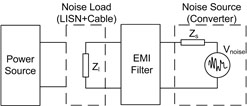Fig. 1. Equivalent circuit to predict EMI filter insertion gain.
This work presents insertion gain predictions for both the differential mode (DM) and the common mode (CM) of an EMI filter. The proposed approach, essentially based on measurements, allows prediction of EMI filter performance for high-complexity converters without time-consuming simulations. The key idea is to use direct measurements to represent a complex system by an equivalent circuit in the frequency domain. The source impedance, which is the more complex part of this equivalent circuit because of its non-linear time variation, is generally masked by external impedance and can be treated as a linear system. Experimental results for insertion gain prediction are included for second-order filters for both DM and CM on a three-phase SiC JFET Vienna/two-level converter.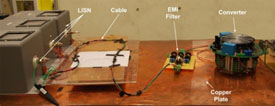Fig. 2. Experimental test setup.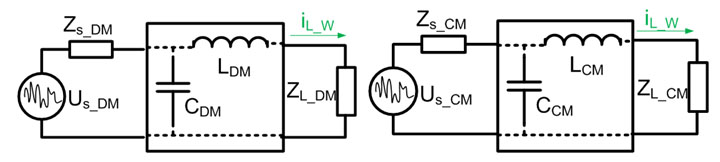Fig. 3. Insertion gain calculation based on the impedance.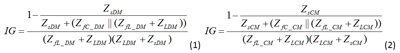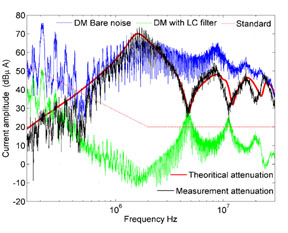Fig. 4. DM insertion gain prediction.Fig. 5. CM insertion gain prediction.
INDUSTRY PARTNERS